Maths-
General
Easy

Question

# If the tangent at the point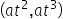on the curve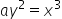meets the curve again at

##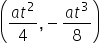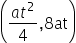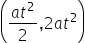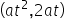Hint:

## The correct answer is:### The given curve is ay2 = x3The point of tangency is (at2, at3)Let the point that tangent intersects again be (as2, as3)We can find the slope of tangent by differentiating the given curve w.r.t xNow, we will use two point form to solve the question.If (x1, y1) and (x2, y2) be two points on the line then the slope is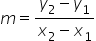For this question, it will be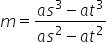We will solve it further and find the value.(s - t) = 0s = tIt means the same point of tangency. We want to find different point.(2s + t) = 0s =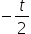So, the point will beThis is the required answer.

For such questions, we should know the formulas to find slope.#### With Turito Foundation.#### Get an Expert Advice From Turito.